# 1.3 Polar Coordinates in the Plane

In polar coordinates a point P is also characterized by two numbers: the distance r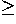0 to a fixed pole or origin O, and the angle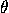the ray OP makes with a fixed ray originating at O, which is generally drawn pointing to the right (this is called the initial ray). The angleis only defined up to a multiple of 360° or 2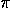. In addition, it is sometimes convenient to relax the condition r0 and allow r to be a signed distance, so (r,) and (-r,+180°) represent the same point (Figure 1).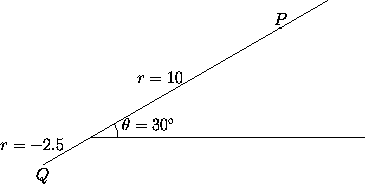Figure 1: Among the possible sets of polar coordinates for P are: (10, 30°), (10, 390°) and (10, -330°). Among the sets of polar coordinates for Q are: (2.5, 210°) and (-2.5, 30°).

### Relations between Cartesian and Polar Coordinates

Consider a system of polar coordinates and a system of cartesian coordinates with the same origin. Assume the initial ray of the polar coordinate system coincides with the positive x-axis, and that the ray=90° coincides with the positive y-axis. Then the polar coordinates (r,) and the cartesian coordinates (x,y) of the same point are related as follows: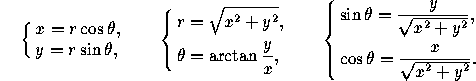Next: 1.4 Homogeneous Coordinates in the Plane
Up: 1 Coordinate Systems in the Plane
Previous: 1.2 Cartesian Coordinates in the PlaneThe Geometry Center Home Page

Silvio Levy
Wed Oct 4 16:41:25 PDT 1995

This document is excerpted from the 30th Edition of the CRC Standard Mathematical Tables and Formulas (CRC Press). Unauthorized duplication is forbidden.